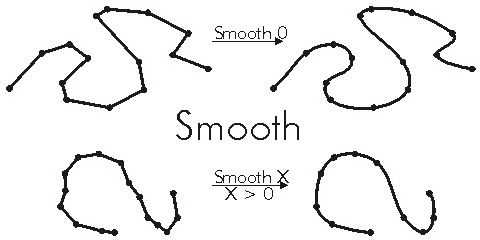This document is archived and information here might be outdated.  Recommended version.

IPolycurve.Smooth Method (ArcObjects .NET 10.7 SDK)
 ArcObjects Help for .NET developers > ArcObjects Help for .NET developers > ArcObjects namespaces > Geometry > ESRI.ArcGIS.Geometry > Interfaces > IP > IPolycurve Interface > IPolycurve.Smooth Method
 ArcGIS Developer Help

# IPolycurve.Smooth Method

Converts this curve into a smooth curve containing only Bezier curve segments.

```[Visual Basic .NET]
Public Sub Smooth ( _
ByVal maxAllowableOffset As Double _
)
```
```[C#]
public void Smooth (
double maxAllowableOffset
);
```
```[C++]
HRESULT Smooth(
double maxAllowableOffset
);
```
```[C++]
Parameters
maxAllowableOffset
maxAllowableOffset is a parameter of type double
```

#### Product Availability

Available with ArcGIS Engine, ArcGIS Desktop, and ArcGIS Server.

#### Description

Converts the Polycurve into a Polycurve containing only BezierCurve segments. If the maxAllowableOffset parameter is zero, each segment of the input Polycurve becomes a separate Bezier curve. If maxAllowableOffset if greater than zero, the polycurve is generalized first by the Douglas-Poiker method using the maxAllowableOffset value. Bezier curves are then created for each of the remaining segments. The created BezierCurve polycurve is an approximation of the original polycurve. At each vertex, the adjoining BezierCurves have complementary tangents which creates a continuous (smooth) transition between segments.

As an alternative, compare IConstructCurve2::ApproximateByBeziers.

#### Remarks